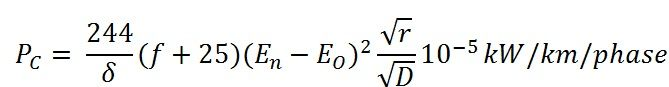# Corona Power Loss

In the previous topic, we learn about the corona effect due to which several losses occur in transmission lines. These losses decrease the efficiency of transmission lines. Out of all the losses the corona power loss is the one which affects most, the proficiency of lines.

The power dissipated in the system due to corona discharges is called corona loss. Accurate estimation of corona loss is difficult because of its variable nature. It has been found that the corona loss under fair weather condition is less than under foul weather conditions. The corona loss under appropriate weather conditions is given below by the Peek’s formula;Where Pc – corona power loss
f – frequency of supply in Hz
δ – air density factor
En – r.m.s phase voltage in kV
Eo – disruptive critical voltage per phase in kV
r – radius of the conductor in meters
D – spacing between conductors in meters

It is also to be noticed that for a single –phase line,

En=1/2×line voltage

and for a three phase line,

En = 1/(√3)×line voltage

Peek’s formula is applicable for decided visual corona. This formula the gives the inaccurate result when the losses are low, and En/Eo is less than 1.8. It is superseded by Peterson’s formula given below;

Pc – corona power loss
f – frequency of supply in Hz
En – voltage per phase
r – radius of the conductor
D – spacing between conductors in meters
Factor F is called the corona loss function. It varies with the ratio (En/Eo). Eo is calculated by the formula given below,

Go – maximum value of disruptive critical voltage gradient in V/m.
mo= irregularity factor

### Factors Affecting Corona Loss

The following are the factors which affect the corona loss:

• Effect of system voltage – The electric field intensity around the conductor depends on the potential difference between the conductors. If the potential difference is high, electric field intensity is also high, and hence corona loss is also high.
• Effect of Frequency – The corona loss is directly proportional to system frequency.
• Effect of Density of Air – The corona loss is inversely proportional to air density factor. The corona loss increases with the decreases in density of air. The corona loss of the hilly area is more than that of the plains because plain have low density of air.
• Effect of Conductor Radius – If the wire area has high surface area, then their surface field intensity is low, and hence corona loss is less.